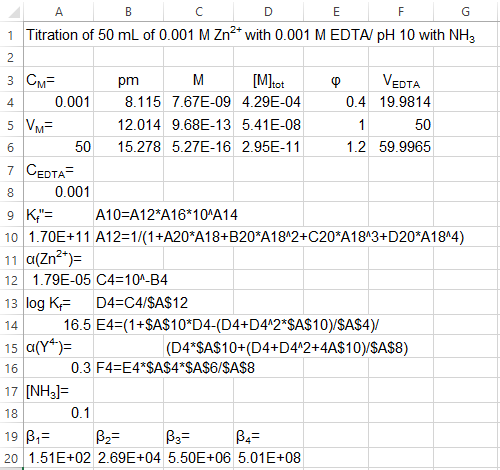×
Get Full Access to Quantitative Chemical Analysis - 8 Edition - Chapter 11 - Problem 11-20
Get Full Access to Quantitative Chemical Analysis - 8 Edition - Chapter 11 - Problem 11-20

×

Auxiliary complexing agent. Use the equation derived in 11-19. (a) Prepare a spreadsheetISBN: 9781429218153 475

Solution for problem 11-20 Chapter 11

Quantitative Chemical Analysis | 8th Edition

• Textbook Solutions
• 2901 Step-by-step solutions solved by professors and subject experts
• Get 24/7 help from StudySoup virtual teaching assistantsQuantitative Chemical Analysis | 8th Edition

4 5 1 305 Reviews
29
0
Problem 11-20

Auxiliary complexing agent. Use the equation derived in 11-19. (a) Prepare a spreadsheet to reproduce the 20-, 50-, and 60-mL points in the EDTA titration of Zn2 in the presence of NH3 in the example on pages 248249. (b) Use your spreadsheet to plot the curve for the titration of 50.00 mL of 5.00 mM Ni2 by 10.00 mM EDTA at pH 11.00 in the presence of a fixed oxalate concentration of 0.100 M. 1

Step-by-Step Solution:

Step 1 of 2

(a)

Given the titration of 50.0 mL ofwithEDTA at pH 10.00 in the presence of the complexing agent 0.10 M. The spreadsheet representing the points 20-, 50-, and 60-mL in the titration is given below:Step 2 of 2

ISBN: 9781429218153

Quantitative Chemical Analysis was written by and is associated to the ISBN: 9781429218153. This textbook survival guide was created for the textbook: Quantitative Chemical Analysis, edition: 8. Since the solution to 11-20 from 11 chapter was answered, more than 234 students have viewed the full step-by-step answer. The answer to “Auxiliary complexing agent. Use the equation derived in 11-19. (a) Prepare a spreadsheet to reproduce the 20-, 50-, and 60-mL points in the EDTA titration of Zn2 in the presence of NH3 in the example on pages 248249. (b) Use your spreadsheet to plot the curve for the titration of 50.00 mL of 5.00 mM Ni2 by 10.00 mM EDTA at pH 11.00 in the presence of a fixed oxalate concentration of 0.100 M. 1” is broken down into a number of easy to follow steps, and 75 words. The full step-by-step solution to problem: 11-20 from chapter: 11 was answered by , our top Chemistry solution expert on 03/16/18, 04:33PM. This full solution covers the following key subjects: . This expansive textbook survival guide covers 55 chapters, and 1224 solutions.

Unlock Textbook Solution